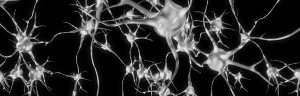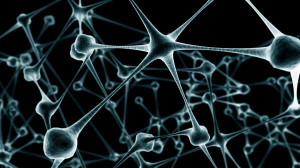## Neural Networks, Part 3: The NetworkWe have learned about individual neurons in the previous section, now it’s time to put them together to form an actual neural network.

The idea is quite simple – we line multiple neurons up to form a layer, and connect the output of the first layer to the input of the next layer. Here is an illustration:

Each red circle in the diagram represents a neuron, and  the blue circles represent fixed values. From left to right, there are four columns: the input layer, two hidden layers, and an output layer. The output from neurons in the previous layer is directed into the input of each of the neurons in the next layer.

We have 3 features (vector space dimensions) in the input layer that we use for learning: $$x_1$$, $$x_2$$ and $$x_3$$. The first hidden layer has 3 neurons, the second one has 2 neurons, and the output layer has 2 output values. The size of these layers is up to you – on complex real-world problems we would use hundreds or thousands of neurons in each layer.

## How to normalise feature vectors

I was trying to create a sample file for training a neural network and ran into a common problem: the feature values are all over the place. In this example I’m working with demographical real-world values for countries. For example, a feature for GDP per person in a country ranges from 551.27 to 88286.0, whereas estimates for corruption range between -1.56 to 2.42. This can be very confusing for machine learning algorithms, as they can end up treating bigger values as more important signals.

To handle this issue, we want to scale all the feature values into roughly the same range. We can do this by taking each feature value, subtracting its mean (thereby shifting the mean to 0), and dividing by the standard deviation (normalising the distribution). This is a piece of code I’ve implemented a number of times for various projects, so it’s time to write a nice reusable script. Hopefully it can be helpful for others as well. I chose to do this in python, as it’s easies to run compared to C++ and Java (doesn’t need to be compiled), but has better support for real-valued numbers compared to bash scripting.

## Neural Networks, Part 2: The NeuronA neuron is a very basic classifier. It takes a number of input signals (a feature vector) and outputs a single value (a prediction). A neuron is also a basic building block of neural networks, and by combining together many neurons we can build systems that are capable of learning very complicated patterns. This is part 2 of an introductory series on neural networks. If you haven’t done so yet, you might want to start by learning about the background to neural networks in part 1.

Neurons in artificial neural networks are inspired by biological neurons in nervous systems (shown below). A biological neuron has three main parts: the main body (also known as the soma), dendrites and an axon. There are often many dendrites attached to a neuron body, but only one axon, which can be up to a meter long. In most cases (although there are exceptions), the neuron receives input signals from dendrites, and then outputs its own signals through the axon. Axons in turn connect to the dendrites of other neurons, using special connections called synapses, forming complex neural networks.

Below is an illustration of an artificial neuron, where the input is passed in from the left and the prediction comes out from the right. Each input position has a specific weight in the neuron, and they determine what output to give, given a specific input vector. For example, a neuron could be trained to detect cities. We can then take the vector for London from the previous section, give it as input to our neuron, and it will tell us it’s a city by outputting value 1. If we do the same for the word Tuesday, it will give a 0 instead, meaning that it’s not a city.